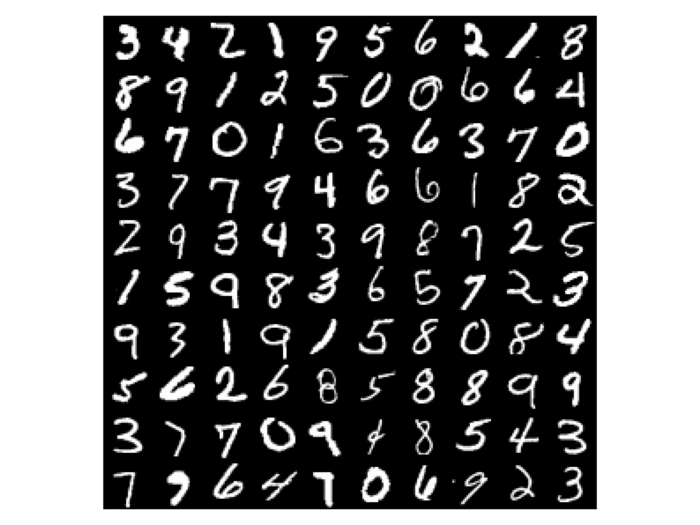# Deep learning training speed with 1080 Ti and M1200

1 min read

I compared the speed of Nvidia’s 1080 Ti on a desktop (Intel i5-3470 CPU, 3.2G Hz, 32G memory) and NVIDIA Quadro M1200 w/4GB GDDR5, 640 CUDA cores on a laptop (CPU: Intel Core i7-7920HQ (Quad Core 3.10GHz, 4.10GHz Turbo, 8MB 45W, Memory: 64G).

The code I used is Keras’ own example (mnist_cnn.py) to classiy MNIST dataset:MNIST dataset
```'''Trains a simple convnet on the MNIST dataset.

Gets to 99.25% test accuracy after 12 epochs
(there is still a lot of margin for parameter tuning).
16 seconds per epoch on a GRID K520 GPU.
'''

from __future__ import print_function
import keras
from keras.datasets import mnist
from keras.models import Sequential
from keras.layers import Dense, Dropout, Flatten
from keras.layers import Conv2D, MaxPooling2D
from keras import backend as K

batch_size = 128
num_classes = 10
epochs = 12

# input image dimensions
img_rows, img_cols = 28, 28

# the data, shuffled and split between train and test sets
(x_train, y_train), (x_test, y_test) = mnist.load_data()

if K.image_data_format() == 'channels_first':
x_train = x_train.reshape(x_train.shape, 1, img_rows, img_cols)
x_test = x_test.reshape(x_test.shape, 1, img_rows, img_cols)
input_shape = (1, img_rows, img_cols)
else:
x_train = x_train.reshape(x_train.shape, img_rows, img_cols, 1)
x_test = x_test.reshape(x_test.shape, img_rows, img_cols, 1)
input_shape = (img_rows, img_cols, 1)

x_train = x_train.astype('float32')
x_test = x_test.astype('float32')
x_train /= 255
x_test /= 255
print('x_train shape:', x_train.shape)
print(x_train.shape, 'train samples')
print(x_test.shape, 'test samples')

# convert class vectors to binary class matrices
y_train = keras.utils.to_categorical(y_train, num_classes)
y_test = keras.utils.to_categorical(y_test, num_classes)

model = Sequential()
model.add(Conv2D(32, kernel_size=(3, 3),
activation='relu',
input_shape=input_shape))
model.add(Conv2D(64, (3, 3), activation='relu'))
model.add(MaxPooling2D(pool_size=(2, 2)))
model.add(Dropout(0.25))
model.add(Flatten())
model.add(Dense(128, activation='relu'))
model.add(Dropout(0.5))
#model.add(Dropout(1))
model.add(Dense(num_classes, activation='softmax'))

model.compile(loss=keras.losses.categorical_crossentropy,
optimizer=keras.optimizers.Adadelta(),
metrics=['accuracy'])

model.fit(x_train, y_train, batch_size=batch_size, epochs=epochs,
verbose=1, validation_data=(x_test, y_test))
score = model.evaluate(x_test, y_test, verbose=0)
print('Test loss:', score)
print('Test accuracy:', score)

```

The result is that 1080 Ti is 3 times faster than M1200:

 M1200 (1 epoch) 1080 Ti (1 epoch) 18s 6s

Receive email notification via email 博客有新内容通知我
 Don't want to miss new papers in your field? Check out Stork we developed:专注医学生物类的论文润色联系他们请用优惠码 STORK4，他们会给你折扣。## Is AI able to write papers?

As researchers, we all have a dream: it would be fantastic if AI could write papers one day! This sounds like a science fiction,...

3 min read

1 min read

23 sec read

## 4 Replies to “Deep learning training speed with 1080 Ti and M1200”

1.Wu Zheng says:

I don’t see M1200 in support list of CUDA. How did you do that?

2.Xu Cui says:

I saw it is listed here at https://developer.nvidia.com/cuda-gpus

3.Daniel Brookshier says:

Was this training or running the recognition?

1.Xu Cui says:

training